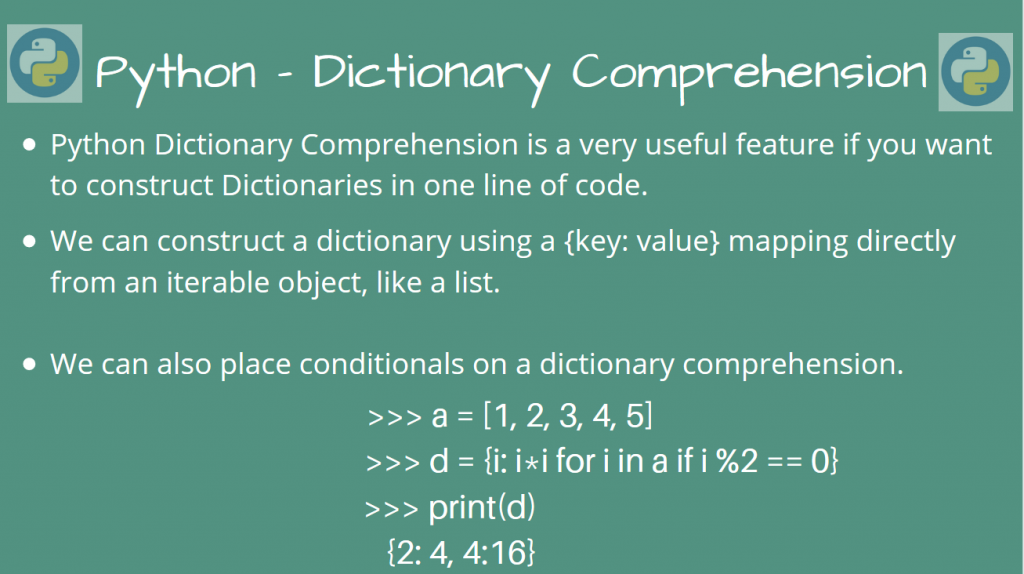# Understanding Python Dictionary ComprehensionIn this article, we’ll take a look at using Python Dictionary Comprehension to create dictionaries easily.

Similar to a List Comprehension, python dictionary comprehension builds a dictionary with our specifications in a single line. This avoids the hassle of creating a dictionary instance and then populating it, using multiple statements.

Let’s look at some of the ways using which we can create dictionaries easily, using illustrative examples!

## Basic Python Dictionary Comprehension

For example, let’s assume that we want to build a dictionary of {key: value} pairs that maps english alphabetical characters to their ascii value.

So, when we call `my_dict['a']`, it must output the corresponding ascii value (97). Let’s do this for the letters `a-z`.

For people unfamiliar with Python code, the normal approach would be to create a dictionary before populating it, like this.

```# Create the dictionary
my_dict = {}

# Now populate it
for i in range(97, 97 + 26):
# Map character to ascii value
my_dict[chr(i)] = i

# Print the populated dictionary
print(my_dict)
```

Output

```{'a': 97, 'b': 98, 'c': 99, 'd': 100, 'e': 101, 'f': 102, 'g': 103, 'h': 104, 'i': 105, 'j': 106, 'k': 107, 'l': 108, 'm': 109, 'n': 110, 'o': 111, 'p': 112, 'q': 113, 'r': 114, 's': 115, 't': 116, 'u': 117, 'v': 118, 'w': 119, 'x': 120, 'y': 121, 'z': 122}
```

While the above code works, we can make this more “Pythonic”, by invoking the Python dictionary comprehension feature, and do this in just one line of code!

For dictionary comprehension, the syntax is similar to this:

```my_diict = {key: func(key) for key in something}
```

or even this

```my_dict = {func(val): val for val in something}
```

Here, `something` can be an iterable, which produces `key` or `val`. The function `func` then maps the key to the value, or vice-versa. You could immediately map the key to the value in one single line, while also creating the dictionary!

In our case, `val` is the variable `i`, while `func(val)` is the function `chr(i)`.

So now, our example can be reduced to the simplified, but still readable code!

```my_dict = {chr(i): i for i in range(97, 97 + 26)}
print(my_dict)
```

Output

```{'a': 97, 'b': 98, 'c': 99, 'd': 100, 'e': 101, 'f': 102, 'g': 103, 'h': 104, 'i': 105, 'j': 106, 'k': 107, 'l': 108, 'm': 109, 'n': 110, 'o': 111, 'p': 112, 'q': 113, 'r': 114, 's': 115, 't': 116, 'u': 117, 'v': 118, 'w': 119, 'x': 120, 'y': 121, 'z': 122}
```

This gives the same output as before! Amazing, isn’t it?

While the above example seems illustrative enough to find the power of dictionary comprehension, can we do more?

The answer is yes, and we could involve even conditionals like `if` and `else` in our dictionary comprehension! Let’s take a look.

## Using Conditionals in Dictionary Comprehension

We can use conditional statements like `if` and `else` to do dictionary comprehension.

Let’s take the first case, where we only want to use an `if` condition.

The syntax for dictionary comprehension will be something like this:

```my_dict = {key: value for key in iterable if condition}
```

In this case, the dictionary will have the mapping only for elements in the iterable where the condition holds.

Suppose we want to construct a dictionary from a list, which maps only even integer numbers to its squares, we can do this, using dictionary comprehension.

```my_list = [0, 1, 2, 3, 4, 5, 6]

my_dict = {i: i*i for i in my_list if i % 2 == 0}

print(my_dict)
```

Output

```{0: 0, 2: 4, 4: 16, 6: 36}
```

Indeed, we only have even numbers in our dictionary, as keys!

Let’s now do a dictionary comprehension using an `else` condition as well!

If the `else` conditional is also needed, we need to modify the syntax for our comprehension. If we want to have the same key which maps to possibly different values, the syntax will look something like this:

```my_dict = {key: (value1 if condition1(value) is True else value2) for key, value in something}
```

Let’s consider building a dictionary using an existing dictionary. The new dictionary will have the value as 0 if it is an odd number. Otherwise, it will simply have the old value, from our previous dictionary.

```old_dict = {'a': 97, 'b': 98, 'c': 99, 'd': 100}

new_dict = {k: (val if val % 2 == 0 else 0) for k, val in old_dict.items()}

print(new_dict)
```

Output

```{'a': 0, 'b': 98, 'c': 0, 'd': 100}
```

## Conclusion

In this article, we learned how we could do Python Dictionary Comprehensions, using some illustrative examples.